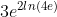## Ln Simplify

Simplify the shown expression:Expand Hint
The logarithm of $$x$$ to the Base $$b$$ is defined by
$$log_b(x)=c$$$where $$b^c=x$$ . Hint 2 Special definitions when $$b=e$$ or $$b=10$$ are: • ln $$x$$ → Base = $$e$$ • log $$x$$ → Base = 10 The logarithm of $$x$$ to the Base $$b$$ is defined by $$log_b(x)=c$$$
where $$b^c=x$$ . Special definitions when $$b=e$$ or $$b=10$$ are:
• ln $$x$$ → Base = $$e$$
• log $$x$$ → Base = 10

According to log properties, the $$2$$ coefficient in front of the natural log can be rewritten as the exponent raised by the quantity inside the log.
$$3e^{2ln(4e)}=3e^{ln[(4e)^2]}$$$Since the natural log has a base of $$e$$ , raising the log by base $$e$$ will eliminate both the $$e$$ and natural log: $$3e^{ln[(4e)^2]}=3(4e)^2$$$
Thus,
$$3(4e)^2=3\times 16e^2=48e^2$$$$$48e^2$$$

Similar Problems from FE Section: Logarithms